Features

# A Non-Integer Power Function on the Pixel Shader

This feature, excerpted from Wolfgang Engel's ShaderX book from Wordware Publishing, presents a simple shader trick that performs a good per pixel approximation of a non-integer power function. The technique works for input values between 0 and 1 and supports large exponents. The presented shader does not require any texture look-up and is scalable, making it possible to spend more instructions in order to decrease the error or to reach greater exponents.

Raising a variable to a non-integer power is an operation often encountered in computer graphics. It is required, for example, to compute the specular term in various shading schemes. It can also be used to produce a smooth conditional function useful in many pixel shaders. In most cases, the input variable falls between 0 and 1 while the exponent is greater than 1 and often quite large.

Like many other techniques, a quality gain can be achieved by computing the function per pixel rather than per vertex. This gain is very noticeable when using large exponents since the function varies a lot and sampling it at each vertex is bound to miss visually important details (see Figure 1).

Therefore, we are particularly interested in finding a way to compute such a function on the pixel shader. Like any pixel shader trick, it is important to minimize the number of textures and blending stages since these are very limited resources. This text presents a simple shader trick that performs a good per pixel approximation of a non-integer power function. The technique works for input values between 0 and 1 and supports large exponents. The presented shader does not require any texture look-up and is scalable, making it possible to spend more instructions in order to decrease the error or to reach greater exponents.

We first consider and analyze two typical techniques used to compute a power function on the pixel shader. We then expose some mathematical background used throughout the text. Finally, we show how the algorithm can be used to perform smooth conditional functions and complex bump-mapped Phong shading. The actual implementation of the approximation as a pixel shader program is discussed in detail.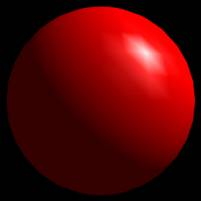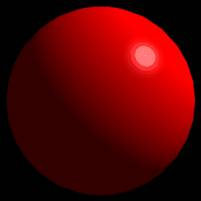Figure 1. Gouraud shading (left) and Phong shading (right)

When confronted with the problem of computing a power function on the pixel shader, two simple techniques come to mind. First, it seems possible to proceed through a 1D texture look-up, and second, applying successive multiplications looks promising.

Texture Look-Up
Linearly interpolated textures can be thought of as piecewise linear functions. In particular, 1D textures with a linear filter are really a function taking a value between 0 and 1 and mapping it onto another value in the same range . This looks promising for our problem since an input between 0 and 1 raised to any power greater than 0 yields a result between 0 and 1.

Listing 1 shows a piece of code that builds a 16-bit monochrome 1D texture of resolution Resi to compute xn:

void ComputePowerTexture( int Resi, double n, unsigned short* Texture )
{
int i;
for( i=0; i
Texture[i] = (unsigned short)( pow( (double)i/Resi, n ) * USHRT_MAX );
}

Listing 1. C Function to create a 1D power texture

Once this texture has been constructed, a simple texture look-up pixel shader can be used to perform the computation, provided that the value to raise to power n is placed in an interpolated texture coordinate. The pixel shader at Listing 2 shows how to apply the power function on the result of a dot product, like it is often required. Note that this code only works for pixel shader versions 1.2 and 1.3. The code for version 1.4 is presented in Listing 3.

ps.1.2

tex t0 ; Texture #0 look-up a vector
texdp3tex t1, t0 ; Use texture coordinates #1 as a 3D vector,
; performs dot product between this 3D vector and t0,
; using the result, look-up power function in 1D texture #1

mov r0, t1 ; emit the result

Listing 2. Code computing a power function using a 1D texture
(pixel shader versions 1.2 and 1.3)

ps.1.4

texld r0, t0 ; Texture #0 look-up a vector
texcrd r1.rgb, t1 ; Load texture coordinates #1 as a 3D vector

dp3 r0, r0, r1 ; Performs dot product between this 3D vector and r0

phase

texld r1, r0.x ; using the result, look-up power function in 1D texture #1

mov r0, r1 ; emit the result

Listing 3. Code computing a power function using a 1D texture

Various problems exist with that particular technique:

• it uses up one texture stage, which may make it unfit to algorithms requiring many textures,
• changing the value of the exponent n requires regenerating the texture, unless a 2D texture is used in which case a limited number of predefined exponents can be used,
• for pixel shaders versions less than 1.4, a 1D texture look-up cannot be applied to intermediate computation results unless multiple passes are used.

This last limitation is often a major drawback since, in usual cases, the power function must be preceded by a vector renormalization (such as done with a cube map) and a dot product. With large exponents, the vector renormalization is especially important. This is due to the fact that the maximum value of a dot product is the product of the length of the two vectors. If one of these is not normalized, the dot product can never reach 1. When raised to a large exponent, a value smaller than 1 will rapidly move toward 0. This translates to visual details being washed out. Figure 2 shows a vector interpolated with and without normalization, followed by a dot product, and then raised to a high power. It is obvious that the detail (for example a specular spot) is washed out in the second version.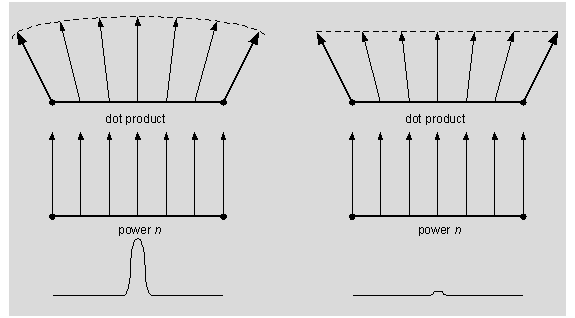Figure 2. Result of not normalizing vectors before applying a power function.

Successive Multiplications
Since raising a value to an integer power simply requires multiplying a value with itself a number of times, it seems possible to approximate a non-integer power function through successive multiplication steps.

For example, the pixel shader at Listing 4 shows how to raise t0 to power 16. Analyzing this scheme indicates that log2 n multiplications are required to raise a variable to the power n, when n is a power of 2:

 ps.1.0 tex t0mul r0, t0, t0 mul r0, r0, r0 mul r0, r0, r0 mul r0, r0, r0 ; r0 = t0 *t0 = t0^2 ; r0 = t0^2*t0^2 = t0^4 ; r0 = t0^4*t0^4 = t0^8 ; r0 = t0^8*t0^8 = t0^16

Listing 4. Power function using successive multiplications

Listing 5 shows a pixel shader that raises t0 to power 31. Analyzing this shows that, in general, for n in the range [2a,2a+1), the algorithm can require 2a multiplications and a temporary variables2.

 ps.1.1 tex t0 mul t1, t0, t0 mul t2, t1, t1 mul t3, t2, t2 mul r0, t3, t3 mul r0, r0, t3 mul r0, r0, t2 mul r0, r0, t1 mul r0, r0, t0 ; t1 = t0^2 ; t2 = t0^4 ; t3 = t0^8 ; r0 = t0^16 ; r0 = t0^16 * t0^8 = t0^24 ; r0 = t0^24 * t0^4 = t0^28 ; r0 = t0^28 * t0^2 = t0^30 ; r0 = t0^30 * t0 = t0^31

Listing 5. Power function with a non-power-of-2 exponent
using successive multiplications

The limitations of this technique are the following:

• only supports discrete changes in the exponent, making it impossible to change the value of n in a continuous fashion,
• requires a lot of instructions for a large exponent,
• requires a lot of instructions and temporary variables for non power of 2 exponents.

These last two problems often limit the usefulness of successive multiplications, since practical exponents have a tendency to be large and are usually not powers of 2.

The Need for a New Trick
Although the 1D texture look-up and the successive multiplications techniques have no limitations in common, both are too restrictive to be really useful in the general case. In particular, none of them is suited to large exponents. In the case of 1D textures, the impossibility to perform a per pixel renormalization before the look-up makes it unsuitable, and for successive multiplications the number of instructions required for large exponents is prohibitive.

The power function approximation technique presented in the rest of the text addresses all of the preceding issues. We therefore think it can often be used as an efficient alternative in pixel shaders that require a power function.

Mathematical Details

If we take xn in the range from 0 to 1 then, for a large enough n, the function is very close to 0 on most of its domain and abruptly goes to 1 when x approaches 1. This is shown in Figure 3 for increasing values of n. This particularity will be the basic concept used for developing our approximation.

What is interesting to note is that the result xn is greater than 1/256 only for values of x greater than 256-1/n. For example, if we take n = 16, the function will be greater than 1/256 only if x is over 0.707, with n = 64 this value becomes 0.917. Since 1/256 is the smallest value displayable on 8 bits per channel hardware, approximating xn with 0 will yield no perceptual error for values of x between 0 and 256-1/n.

Now, if we look at xn for input values greater than 256-1/n, we can see that it looks pretty much like a scaled and offset power function of a lower degree. Figure 4 shows the function x16 being approximated by x4 scaled and offset horizontally to reach 0 when x = 256-1/16.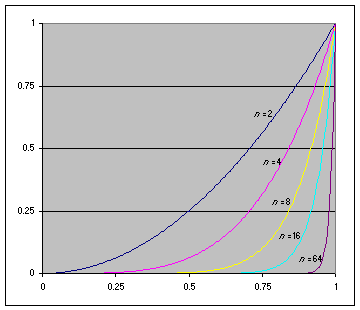Figure 3. Shape of a power function with increasing exponents n = 2, 4, 8, 16, 64.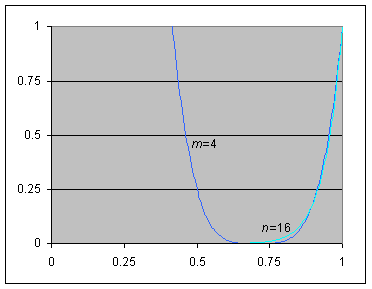Figure 4. Approximating the right part of a power function with a lower degree exponent m.

Therefore, using the null function from 0 to 256-1/n and correctly scaling a lower degree power function for the rest of the range seems to constitute an adequate approximation. Say our approximating function uses an exponent m, a scaled and offset version of the function can be written as (Ax + B)m, where A is the scale and B is the offset. Now, naturally, we want this approximating function to be equal to 1 when x is 1. Also, we want it to reach 0 when x = 256-1/n. We can therefore solve for A and B. We find that A = 1 / ( 1 - 256-1/n ) and B = 1 - A. The approximating function can be written as: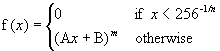By noting that x < 256-1/n if and only if Ax + B < 0, the above function can be rewritten more concisely as:

f(x) = max(Ax + B, 0)m

Note that we will always consider m £ n in the rest of the text. This is because we want the approximating function to have a lower degree than the original.

This technique can now be used with real examples. Figure 5 shows the result of approximating xn with max( Ax + B, 0 )m for n = 16 and m = 4. In this case, A and B are computed as described earlier and their value is A = 3.4142 and B = -2.4142. The graph displays both the original and approximated curves. The normalized error function is also plotted in order to show how the error is distributed to the left and right of the point where both curve crosses.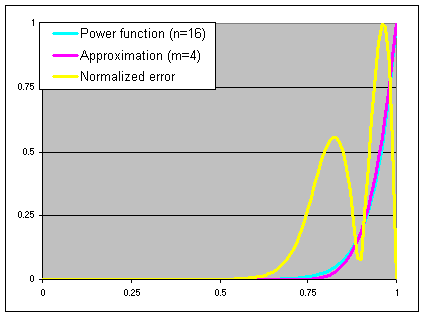Figure 5. Approximation of a power function and normalized error.

A first analysis shows that the approximation gives good results for these values of A, B, n and m. However, if we look more closely at the graph we can notice that the error is not distributed equally on each side of the crossing point. This leads us to think that the maximal approximation error could be lowered by adjusting A and B in order to move the crossing point.

In fact, for arbitrary values of n and m, the technique we've described to select A and B doesn't give any guarantee on the maximal approximation error. In practice, however, it may be suited to many applications.

In order to optimize the approximation error, one should seek values of A and B for which the maximal error on the left and right side are equal. We solved this problem using the numerical approach presented in Listing 6 and described next.

First we need a function that, given approximate values for A and B, is able to compute the maximal error on the left and right side of the crossing point. To do so, we simply find the zero of the derivative of the error function on the left and right sides and evaluate the error function at these points. This is accomplished by the function EvaluateMaxError.

Then, we perform a binary search, changing our guess values for A and B in order to move the crossing point. When the left error is greater than the right error, the crossing point is moved to the left, otherwise it is moved to the right. This is accomplished by the function FindAB.

This algorithm guarantees that we will pick values of A and B that minimize the maximal approximation error for any n and m, provided that 1£ m £ n.

Listing 6. C code to determine optimal scale and offset for some approximation

const double Epsilon = 0.0001;
const double ThresholdError = 0.0001;

// Find values for A and B minimizing the maximal error given n and m
void FindAB( double n, double m, double &A, double &B )
{
double k0 = 0;
double k1 = 1;
double k;
double ErrorLeft, ErrorRight;

// Binary search for optimal crossing point
do
{
k = (k0 + k1)/2.0;
A = ( pow( k, n/m ) - 1.0 ) / ( k - 1.0 );
B = 1.0 - A;

EvaluateMaxError( n, m, A, B, k, ErrorLeft, ErrorRight );

if( ErrorLeft < ErrorRight )
k0 = k;
else
k1 = k;

} while( fabs( ErrorLeft - ErrorRight ) > ThresholdError );
}

// Evaluate the maximal error to the left and right of the crossing point
// for given values of A and B
// The crossing point k is given to reduce computation
void EvaluateMaxError( double n, double m, double A, double B, double k,
double &ErrorLeft, double &ErrorRight )
{
double x;

double DerErr;

// Find maximal point of the error function on the left of the crossing point
double x0 = 0;
double x1 = k;
do
{
x = (x0 + x1)/2.0;

DerErr = DerError( x, n, m, A, B );
if( DerErr < 0 )
x0 = x;
else
x1 = x;
} while( fabs( x0-x1 ) > Epsilon );

// Evaluate the error at that point
ErrorLeft = fabs( Error( x, n, m, A, B ) );

x0 = k;
x1 = 1;

// Find maximal point of the error function on the right of the crossing point
do
{
x = (x0 + x1)/2.0;

DerErr = DerError( x, n, m, A, B );
if( DerErr > 0 )
x0 = x;
else
x1 = x;
} while( fabs( x0-x1 ) > Epsilon );

// Evaluate the error at that point
ErrorRight = fabs( Error( x, n, m, A, B ) );

}

// Evaluate the approximation function
double Approx( double x, double m, double A, double B )
{
if( x < -B/A )
return 0;
return pow( A * x + B, m );
}

// Evaluate the derivative of the approximation function
double DerApprox( double x, double m, double A, double B )
{
if( x < -B/A )
return 0;
return A*m*pow( A * x + B, m-1 );
}

// Evaluate the error function
double Error( double x, double n, double m, double A, double B )
{
return Approx( x, m, A, B ) - pow( x, n );
}

// Evaluate the derivative of the error function
double DerError( double x, double n, double m, double A, double B )
{
return DerApprox( x, m, A, B ) - n*pow( x, n-1 );
}

Using this algorithm with the previous example, where n = 16 and m = 4, yields A = 3.525 and B = -2.525. This is illustrated in Figure 6. It can be seen that the error is equally distributed to the left and right of the crossing point, indicating that the maximal error has been minimized.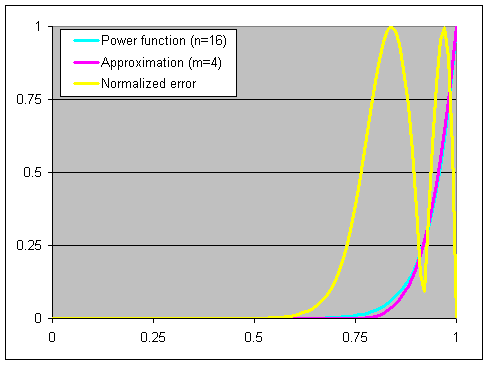Figure 6. Approximation of a power function using optimized scale and offset.

It should be noted that, by selecting A and B through the algorithm of Listing 6, we lose the property that the max( Ax+B, 0 )m is equal to 0 only for values of xn < 1/256. However, this doesn't hurt our approximation since the maximal error has been lowered.

Table 1 shows the maximal approximation error for typical values of n and m. It also shows the optimal values of A and B for these values.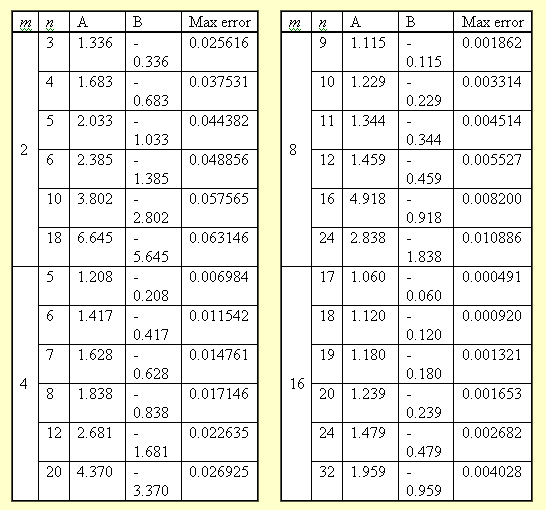Table 1. Scale, offset and maximal error
for typical approximations.

Power Function on the Pixel Shader

Approximating a power function on the pixel shader requires us to translate the preceding mathematical reasoning into the pixel shader assembly language. Doing so requires us to compute the function max( Ax+B, 0 )m through the set of available microcode instructions. We also need a way to specify the variables present in this equation, namely A, B, m and x.

We can rule out a number of these variables easily: the input variable x will simply be stored in a general-purpose register and the exponent m will be decided in advance. For variables A and B we will consider two scenarios. At first, they will be fixed ahead of time and their content will be stored in constant registers. In the second scenario, we will show how A and B can be modified dynamically on a per pixel basis.

Constant Exponent
Let's first study the case where A and B do not change per pixel. In this scenario, A is placed in the constant register c0 while B is placed in c1. This means that the exponent n being approximated is constant as long as c0 and c1 remain unchanged.

Now we need to compute max( Ax+B, 0 )m. First, the max( …, 0) function is taken care of using the _sat modifier available on the pixel shader. Then, we pick m as a power of 2 selected to approximate with enough precision the target exponent n. We then perform a bias and scaling with mad, followed by log2 m self-multiplications with mul. The result is the pixel shader of Listing 7, where the power function is applied to each element of the vector r0. It should be noted that log2 m + 1 is equal to the number of pixel shader stages required. Therefore, in an actual shader, the number of free instructions could limit m.

ps.1.0

... ; Place input value x into r0

ad_sat r0, c0, r0, c1 ; r0 = max( Ax + B, 0 )
mul r0, r0, r0 ; r0 = max( Ax + B, 0 )^2
mul r0, r0, r0 ; r0 = max( Ax + B, 0 )^4

.
. ; repeat (log2 m) times the mul instruction
.
mul r0, r0, r0 ; r0 = max( Ax + B, 0 )^m

Listing 7. Basic code for approximating a power function

There is an important problem with the previous pixel shader. In fact, since all constant registers must be between -1 and 1, we have a very limited range of values for A and B. Table 1 shows that, for typical values of n and m, A is always greater than 1. Therefore, in practice, the proposed pixel shader is invalid.

To limit ourselves to scale and offset values in the allowed range, we first rewrite the approximation function as follow:

max( Ax+B, 0 )m = k max( (Ax+B)k-1/m, 0 )m = k max( A'x+B', 0 )m

where we introduced variables A' and B' defined as:

A' = Ak-1/m B' = Bk-1/m

Therefore, A and B can be written:

A = A'k1/m B = B'k1/m

From these last two relations we can see that, with A' and B' between -1 and 1, we can obtain values of A and B between -k1/m and k1/m. Given that k is greater than 1, this translates to an increased range for A and B.

The pixel shader lets us compute k max(A'x+B', 0)m with k greater than 1 through its multiply instruction modifiers _x2 and _x4. If we take the program of Listing 7 and apply such modifiers to some or each of the mad or mul instructions, we will get a k greater than 1.

It is possible to compute k given a sequence of multiply instruction modifiers. This is performed by the function ComputeK in Listing 8. Before calling any function in this listing, make sure to correctly initialize the global array Multiplier so that it contains the correct multiply instruction modifier for each instruction (either 1, 2 or 4). If we want to know which value of A' and B' correspond to some values of A and B, we can use the computed k and the equation presented earlier, this is done by the function ComputeAB.

We go in the opposite direction and find the maximal values for A and B given k and m, as performed by the function MaxAB. This result can then be converted in a maximal value for n, as computed by MaxN.

// Table containing multiply instruction modifier for each instruction (1, 2 or 4)
int Multiplier[] = { 4, 4, 4, 4, 4, 4, 4, 4 };

// Compute value of k at instruction i given a table of multiply instruction modifiers
double ComputeK( int i )
{
if( i == 0 )
return Multiplier[i];

double Temp = ComputeK( i-1 );
return Multiplier[i] * Temp * Temp;
}

// Compute values of A' and B' given A and B and a multiplier table
// LogM: log of m in base 2 (number of instructions - 1)
void ComputeApBp( int LogM, double A, double B, double &APrime, double &BPrime )
{
double Temp = 1.0/MaxAB( LogM ); // Note that k -1/m = 1/MaxAB

APrime = A * Temp;
BPrime = B * Temp;
}

// Compute maximum absolute values for A and B given some m and a multiplier table
// LogM: log of m in base 2 (number of instructions - 1)
double MaxAB( int LogM )
{
double m = pow( 2.0, LogM ); // Find the value of m
double K = ComputeK( LogM ); // Compute K
return pow( K, 1.0/m );
}

// Compute maximum possible exponent given some m and a multiplier table
// LogM: log of m in base 2 (number of instructions - 1)
double MaxN( int LogM )
{
double m = pow( 2.0, LogM ); // Find the value of m
double Max = MaxAB( LogM );

double A;
double B;

double n0 = m; // Lower bound for maximal exponent
double n1 = 5000; // Upper bound for maximal exponent
double n;=

do
{
n = (n0 + n1)/2.0;

FindAB( n, m, A, B );

if( fabs(A) > Max || fabs(B) > Max )
n1 = n;
else
n0 = n;

} while( fabs( n0 - n1 ) > Epsilon );

return n;
}

Listing 8. C code for computing corrected scale and offset A' and B'

It can be seen that the maximum value n is obtained when the modifier _x4 is used for each instruction in the code. Given that the value for A' and B' are stored in constant registers c0 and c1 respectively, the pixel shader at Listing 9 performs this approximation.

ps.1.0

... ; Place input value x into r0

mad_x4_sat r0, c0, r0, c1 ; r0 = 4 * max( A'*x + B', 0 )
mul_x4 r0, r0, r0 ; r0 = 4 * (4*max( A'*x + B', 0 ))^2
mul_x4 r0, r0, r0 ; r0 = 4 * (4*(4*max( A'*x + B', 0 ))^2)^2
.
. ; repeat (log2 m) times the mul
.
mul_x4 r0, r0, r0 ; r0 = 4^(2m-1) * max( A'*x + B', 0 )^m

Listing 9. Corrected code for approximating a power function

Table 2 shows the maximal range for A and B and the maximal exponents n that can be obtained with the previous shader for various values of m.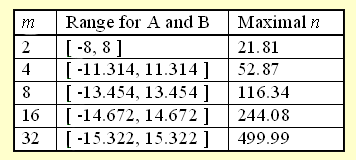Table 2. Maximal values of A, B and n available depending
on approximation exponent m
.

Naturally, if for a given m we want to limit ourselves to exponents smaller than the maximal n listed in this table, we can remove some _x4 modifiers or replace them by _x2. When doing this, we figure the new maximal n by using the MaxN function with an updated Multiplier array.

Not using _x4 modifiers at each instruction is often a good idea since it can help reduce the numerical imprecision often present in pixel shaders. This lack of precision is mostly noticeable for small values of n since they translate to values of c0 and c1 close to zero. Such small numbers may suffer from an internal fixed-point representation and yield visual artifacts.

Per Pixel Exponent
Until now we have considered that the exponent to approximate n was fixed per pixel and could be stored in constant registers. However, being able to vary the exponent based on the result of a texture look-up is sometimes required. The proposed pixel shader trick can be extended to support that. To do so we must decide ahead of time a value for m and a sequence of multiplication modifiers. These must be chosen in order to cover all possible values of n, for n> m.

Once we have picked these values we simply take a texture image of the desired n and translate each texel to their corresponding value of A. The texels are then translated to A' through a multiplication by k-1/m. This time, however, instead of storing the result in a constant register, we update the texture. The program in Listing 10 executes the process.

// Translates a texture of exponents n into values that
// can be directly used by the pixel shader
// Texture: monochrome texture in custom format
// ResX: X resolution of the texture
// ResY: Y resolution of the texture
// LogM: log of m in base 2 (number of instructions - 1)
void TranslateTexture( double** Texture, int ResX, int ResY, int LogM )
{
double A;
double APrime;
double Dummy;
double m = pow( 2.0, LogM ); // Find the value of m

for( int i=0; i
for( int j=0; j
{
FindAB( Texture[i][j], m, A, Dummy );
APrime = A/MaxAB( LogM ); // Compute A'. Note that k -1/m = 1/MaxAB
assert( fabs(APrime) <= 1 ); // If assert fails select another m
// or change the multipliers

Texture[i][j] = APrime; // Update the texture
}

}

Listing 10. C code to generate an approximation texture
based on an exponent texture

Once such a texture has been generated and placed in texture stage 0, we can easily extract A' inside a pixel shader. We can also extract B' by recalling that B = 1 - A. Since B' is the result of multiplying B by k-1/m, we can write B' = (1 - A)k-1/m = k-1/m - A'. Since m is fixed, we store k-1/m in constant register c0 and perform a simple subtraction to extract B'.

The pixel shader of Listing 11 approximates a power function with n varying per pixel. This shader uses modifiers _x4 for each instruction, the texture and the constant register c0 should therefore be generated accordingly.

 ps.1.0 tex t0 ... sub r1, c0, t0 mad_x4_sat r0, t0,r0,r1 mul_x4 r0, r0, r0 mul_x4 r0, r0, r0 . . . mul_x4 r0, r0, r0 ; Sample the value c0 for approximation ; Place input value x into r0 ; Compute B' = 1/MaxAB(LogM) - A' ; r0 = 4 * max( A'*x + B', 0 ) ; r0 = 4 * 16 * max( A'*x + B', 0 )^2 ; r0 = 4 * 16 * 256 * max( A'*x + B', 0 )^4 ; repeat (log2 m) times the mul ; r0 = 4^(2m-1) * max( A'*x + B', 0 )^m

Listing 11. Code for approximating a power function with
an exponent n varying per pixel

This shader seems to show that one extra instruction is required to handle an exponent n varying per pixel. However, this is only true if we cannot spare additional texture space. In the case where a texture or a texture component is still available, we could precompute the value of B' and store it there. However, we believe that textures are often a more limited resource than pixel shader instructions, this is why we suggest you use the approach presented above.

As a last remark, we can note that the power function often only needs to be computed on a scalar. Therefore, provided that the input value x is placed in the alpha channel, the pixel shader can co-issue instructions. In such a case, we can also limit our usage of constant registers by using only the alpha channel of the constants. This technique is applied in the pixel shaders presented in the rest of the text.

Applications

The nature of the power function makes it very interesting for a number of applications in computer graphics. Any effect that should be visible at some point and fade-out more or less rapidly for neighboring points can benefit from such a function. In this section we will present two techniques that require a per pixel power function: smooth conditionals and specular Phong shading.

Smooth Conditional Function

Let's first start by studying the conditional instructions available in the pixel shader. We take cmp because it behaves similar to cnd while being more general:

cmp dest, src0, src1, scr2

This instruction loads dest with src1 or src2 depending whether src0 is positive or not. If we consider this instruction with values of src1 = 1 and src2 = 0, we obtain the function illustrated at Figure 7.

Since the conditional instructions can only take one of two values, they often produce jaggy-edge artifacts. In fact, some neighboring pixels end up having very different colors because they sit on the threshold of the condition.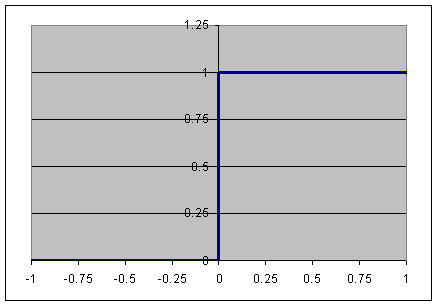Figure 7. Standard conditional function.

To overcome this problem, we can use the function illustrated at Figure 8. We call such a function a smooth conditional because it smoothly and rapidly goes from one value to the other as some threshold is crossed.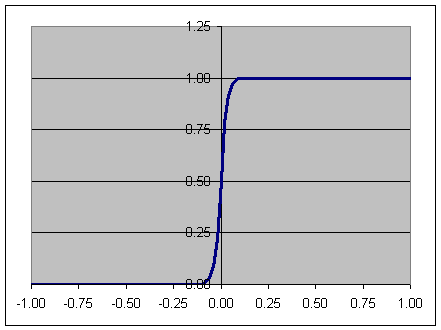Figure 8. Smooth conditional function.

The function illustrated in Figure 8 corresponds to the following mathematical formula: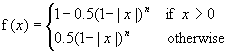This formula can be computed on the pixel shader using the code of Listing 12. This code is similar to the one of Listing 9. The difference is that it uses two extra cmp instructions, one for the absolute value and one for the condition x>0. Also, note that the last mul has a _x2 multiply instruction modifier although it should be considered as a 4 in the Multiplier table when generating A' and B'. This is done in order to account for the multiplicative factor in the term 0.5 (1 - |x|)n appearing in the formula.

 ps.1.2 ; c2 = -1, -1, -1, -1 ... cmp r1, r0, r0, -r0 mad_x4_sat r1, c0, 1-r1, c1 mul_x4 r1, r1, r1 mul_x4 r1, r1, r1 . . . mul_ x2 r1, r1, r1, c2 cmp r0, r0, 1-r1, r1 ; Used for the sign function ; Place input value x into r0 ; r1 = |x| ; r1 = k0 * max( A'*(1-|x|) + B', 0 ) ; r1 = k1 * max( A'*(1-|x|) + B', 0 )^2 ; r1 = k2 * max( A'*(1-|x|) + B', 0 )^4 ; repeat (log2 m) times the mul ; r1 = 0.5 * k * max( A'*(1-|x|) + B', 0 )^m ; r0 = 1 - 0.5*(1-|x|)^n if x > 0 ; r0 = 0.5*(1-|x|)^n otherwise

Listing 12. Code to perform a smooth conditional function
(pixel shader versions 1.2 and 1.3)

We can use the above code to build a smooth conditional selection between two arbitrary values src1 and src2. To do so, we simply add a lrp instruction at the end of the previous pixel shader. This linear interpolation is made to map 0 to src1 and 1 to src2. The result is a function that mimics the standard cmp in a smooth fashion.

The presented code requires a lot of instructions; however we can co-issue them if we want the smooth conditional to act only on the alpha channel. Moreover, the simplified asymmetric shape of Figure 9 often constitutes a good enough smooth conditional for x>0. Given that the input x is saturated (greater or equal to 0) then this function can be expressed as 1-(1-x)n.
This is computed with minimal change to the code of Listing 9. We simply need to use the invert source modifier at the input and output.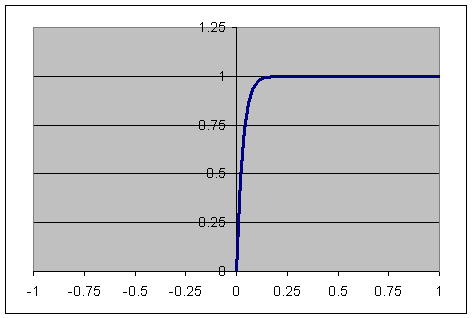Figure 9. Simplified smooth conditional function.

Other variations on the smooth conditional pixel shader code allows for various other arithmetic tests. In fact, the simple power function xn can be considered as the smooth conditional for x>1.

A problem that may arise with pixel shaders is that sometimes per pixel effects needs to occur only within a given volume. For example, say we have a magical spell that affects every pixel within a given radius of the casting point. If we want that spell to turn on a specific per pixel effect, we need to find if each pixel is inside the spell radius using some pixel shader instructions. This can be done easily with a standard conditional instruction. However, if we do that, then we will most probably suffer from the jaggy-edge artifacts mentioned earlier. This is therefore a good place where the smooth conditional function could be used.

So let's see how we can build a pixel shader that equals 1 for pixels within some radius r of a point P and smoothly goes to 0 for pixels outside this radius. First, for each vertex, we'll require a vector R that joins point P to the vertex. This vector needs to be scaled so that its length equals 1 for a vertex that is exactly at distance r from P. We place the vector P in texture coordinates 0. We will directly use this vector, therefore no texture needs to be bound to stage 0.

We then select the approximation exponent m for the power function used in the smooth conditional. In the presented example, we take m = 4. The multiply instruction modifier _x4 was used with each instruction. Since we use a fixed exponent n for the shader, we place A' and B' in constant registers c0 and c1.

The pixel shader of Listing 13 uses this technique to apply a simple diffuse lighting equation to all pixels within some radius of a given point. The diffuse color is placed in interpolated color v0, texture 1 contains the decal texture to apply and texture 2 holds an extra diffuse light map. A constant ambient factor is stored in constant register c2. More complex effects could use the pixel shader same trick in order to limit themselves to a volume. Also, more complex volumes could be devised by applying some per vertex or per pixel process on P.

ps.1.0

; c0 ; A'
; c1 ; B'
; c2 ; Ambient factor
; c7 = 1, 1, 1, 1 ; Uniform white, used in shader

texcoord t0 ; Pass on vector P
tex t1 ; Fetch decal texture

; Pixel ops
dp3_sat r0, t0, t0 ; r0 = max(0, P.P) = |P|^2

add r0.rgb, v0, t2 ; Compute diffuse lighting by adding v0 and t2
+mad_x4_sat r0.a, c0, r0, c1 ; r0.a = k0 * max( A'*x + B', 0 )

mul r0.rgb, r0, t1 ; Apply diffuse lighting to decal
+mul_x4 r0.a, r0, r0 ; r0.a = k1 * max( A'*x + B', 0 )^2

+mul_x4 r0.a, r0, r0 ; r0.a = k * max( A'*x + B', 0 )^4

mul r0.rgb, 1-r0.a, r0 ; 1-r0.a = SmoothConditional( |P|^2 < 1 )
; Multiply by result of shading
+mov r0.a, c7 ; Clear alpha

Listing 13. Code to perform volume bounded pixel shader lighting

One of the major problems of per pixel lighting resides in computing the specular component of the final color. This component is due to the reflection of the light itself on a shiny material. It is easy to see that the reflection of a point light on a perfectly shiny sphere is a single point. Unfortunately, very few surfaces are perfectly shiny. To account for non-perfect reflections, sometimes called dull reflection, Phong (and Warnock before him) introduced a very popular model. This model relies on the use of a power function where the exponent depends on the shininess of the material.

Pixel shader algorithms to perform Phong shading have been described before. However, they performed the per pixel power function using the usual techniques described at the beginning, therefore suffering from the problems of such techniques. This often leads to multi-passes algorithms or it reduces the control over the shininess exponent.

We will now show how, using the power function algorithm exposed earlier, we can perform realistic per pixel Phong shading including a normal map, a color diffuse texture map, a color specular texture map, and a shininess map.

Phong Equation with Blinn Half-Vector
First, we need to express our shading model mathematically. We refer you to computer graphics textbooks for more information on the equations presented in this section.

Before we can express this equation, we need to detail the variables that are needed. First, the shading depends upon the light direction, described by unitary vector L. We also need to know the surface normal at the shading point, noted N. Finally, we need to use some vector to compute the specular component. Like it is often the case for per pixel shading, we do not directly use the Phong equation. Instead we take the Blinn half-vector H, that is the unitary vector falling directly between the view direction V and the light direction L. Figure 10 illustrates these vectors.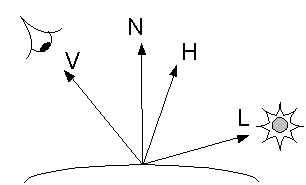When using normal mapping, we distinguish between the perturbed normal used for lighting, noted N' and the real surface normal noted N.

The scalar values that are needed for the computation are the ambient, diffuse and specular coefficients noted respectively MAmbc, MDiffc, and MSpecc for the material, and LAmbc, LDiffc, and LSpecc for the light. Here the index c is R, G or B to indicate one of the color components.

The shading equation can now be written as: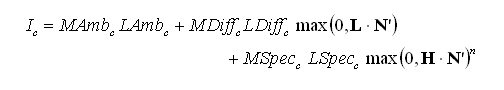Expressing the Inputs
The Phong equation has a number of inputs that we need to be able to pass down to the pixel shader. We will first make a number of assumptions that reduces the number of inputs required for the above function.

First, we suppose that the light ambient, diffuse and specular coefficients do not vary from one pixel to the next. This means that the algorithm proposed cannot handle slide-projectors or other kinds of textured lights.

We also suppose that the material ambient coefficients do not vary arbitrarily from pixel to pixel. Instead, these are linked to the material diffuse coefficients using the equation MAmbc = KAmbc *MDiffc, with KAmbc constant within the shader. The equation states that the ambient color is always the same as the diffuse color up to some constant. This is not a very limiting assumption since this relationship between material ambient and diffuse coefficients is often witnessed in practice.

The three coefficients MDiffc and exponent n can vary from pixel to pixel and therefore need to be expressed in a four-component, 2D texture map. Recall that we do not store n directly, instead we place the corresponding value A' in the texture's fourth component. Coefficients MSpecc can also vary at each pixel and can be placed in a separate three-component, 2D texture. These coefficients act as a color gloss map, effectively an extension of the traditional single-component specular gloss map.

The perturbed normal N' is expressed as a tangent-space normal map. We therefore use a 2D texture map containing color-encoded normalized vectors that can be accessed using a single 2D texture look-up. We refer the reader to other real-time shading texts to learn more on tangent space normal maps.

To effectively use a tangent-space normal map in our lighting equation, we need to have a normal map representation of the light vector L and halfway vector H. As discussed earlier, the halfway vector needs to be renormalized per pixel; otherwise important visual artifacts will occur when the power function is applied. We therefore interpolate H through a texture coordinate and use a cube map look-up to renormalize it. A renormalization cube map, as illustrated in Figure 11, contains a color-encoded vector in each texel corresponding to the unitary vector pointing in the direction of that texel.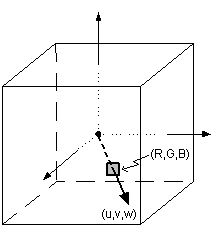Figure 11. Renormalization cube map.

Once the light vector is only used in linear computations, not renormalizing it has almost no impact on the visual result. Therefore, we skip per pixel renormalization in order to make the best usage out of our texture resources. This means that we can store the light vector L in an interpolated color.

Let's put all this together into a single pixel shader. First, each texture stage and interpolated color is assigned as follows: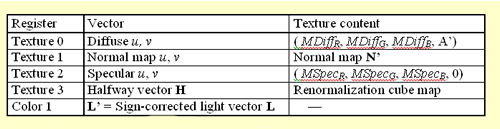It should be noted that, since interpolated colors do not support negative values, we place the sign corrected light vector in color 0. This can be written as L' = 0.5(L + (1,1,1) ).

We need to have access to KAmbc and LAmbc that are constant from one pixel to the next. Due to the nature of the equation, we can precompute the product of these coefficients. Therefore, we store the resulting vector (KAmbR*LAmb, KAmbG*LAmbG, KAmbB*LAmbB ) in the first three components of constant register c0.

We also need the values of constant coefficients LDiffc and LSpecc. These are stored in the first three components of registers c1 and c2, respectively.

Finally, we need to pick the approximation exponent m used for the power function. In our example, we use m = 8. We also use _x4 as the multiply instruction modifier for each instruction of the power function approximation. Since we wish to use a value of n varying per pixel, we must precompute k-1/m = 0.074325. We store this value in the alpha channel of constant register c0. Looking at Table 2, we find that the maximal n that can be achieved is 116.34. Also, using the function TranslateTexture of Listing 10 we can convert per texel values of n into values of A' to be placed in the alpha component of texture 0.

ps.1.1
; v1 RGB: light vector (L') (sign corrected)
; c0 RGB: ambient light (Kamb*Lamb), A: 1/MaxAB(logM) = 0.074325
; c1 RGB: diff light (Ldiff), A: 0
; c2 RGB: spec light (Lspec), A: 0

tex t0 ; diffuse texture (Mdiff) + A' (exponent')
tex t1 ; normal map (N')
tex t2 ; specular texture (Mspec)
tex t3 ; normalised halfway vector (H)

dp3_sat r0.rgb, v1_bx2, t1_bx2 ; L'.N'
+ sub r0.a, c0.a, t0.a ; B'

dp3_sat r1, t3_bx2, t1_bx2 ; H.N'

mad r0.rgb, r0, c1, c0 ; Kamb*Lamb + Ld*L'.N'
+ mad_x4_sat r1.a, t0.a, r1.a, r0.a ; p0 = k0*max(A'x+B', 0)

mul r1.rgb, t2, c2 ; Ms*Ls
+ mul_x4 r1.a, r1.a, r1.a ; p1 = p0*p0

mul_x4 r1.a, r1.a, r1.a ; p2 = p1*p1
mul_x4 r1.a, r1.a, r1.a ; p = p2*p2 = H.N'^n

mul r1.rgb, r1, r1.a ; Ms*Ls*H.N'^n
mad r0, r0, t0, r1 ; Ma + Md*Ld*L'.N' + Ms*Ls*H.N'^n

Listing 14. Code for Phong shading with various features
(pixel shader versions 1.1, 1.2 and 1.3)

Summary

We have presented a method of approximating a non-integer power function on a pixel shader by using as few texture stages as possible and gracefully degrading in accuracy depending on the desired exponent and available number of blend stages. Furthermore, the technique can be used for single or multiple channels, thus adapting nicely to individual shader requirements.

The method has several advantages over traditional exponentiation techniques that use either a texture look-up or a series of sequential multiplications. Texture look-ups are only accurate for pixel shader versions 1.4 and greater, and even then will require two phases. Sequential multiplications need a large number of stages to compute high power-of-two exponents and even more for non-power-of-two. Additionally, since the multiplications are inherently uniform during the entire shader, they do not allow for a smooth variation in power.

A couple of applications were suggested, and Phong shading in particular is covered in the results below. We believe such a useful technique can be applied to many other algorithms that require a power function, especially considering that it can be abstracted to any effect requiring a sharp yet smooth transition, such as a spotlight's cone falloff.

The per pixel variation of the exponent, which is a handy extension to the basic principle, can provide important visual cues for surfaces whose specularity varies, such as for a material including both metallic and organic features. Its main disadvantages are that m constrains the lower bound of the specular exponent n, as explained in the mathematical details section, and that one component of a texture must be used to encode the exponent. The latter, however, is expected of any technique that varies the exponent per pixel.

The shading code of Listing 14 was applied to red spheres with different properties, such as faceted per pixel exponent maps, wrinkled normal maps, and orange specular maps. The results can be seen in Figure 12. Due to the many iterative multiplications, there is a large accumulation of error that manifests itself as banding. Generally speaking, the greater the exponent, the more banding will be evident, however, this is mostly noticeable on smooth surfaces, such as those expressed with uniform normal maps. The banding artifacts are less significant when using normal maps that contain some perturbation because the specular falloff is quite sharp due to the abrupt changes in the normal. Therefore, visual artifacts are reduced as detail in the normal map is increased.

Banding can also result from reduced instruction counts. If additional instructions are available, we recommend using them to maximize precision. Note that since the Phong shading algorithm presented only requires exponentiation of a single component, the instructions easily fit into the scalar pipeline of the pixel shader, which reduces the number of dedicated stages. The shader code of Listing 14 consumes two stages purely for exponentiation purposes, but instructions in the vector pipeline can be co-issued at these stages if desired.

For example, the specular texture can be removed if the diffuse and specular materials are represented by a single texture. We can then use a cube map at that stage to represent the surrounding environment, even encoding a Fresnel term in the remaining colour register. The math computations can easily be accommodated within the two remaining vector instructions.

Finally, the images in Figure 12 were rendered on hardware with 8 bits of fractional precision. Other hardware is available which has more than 8 bits of fractional precision and will suffer less from the banding artifacts.

We hope you found this trick helpful and that you will find many more uses for an approximating power function in your shading endeavors.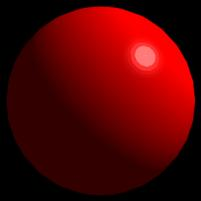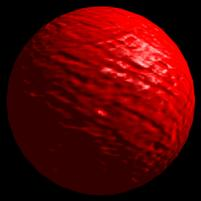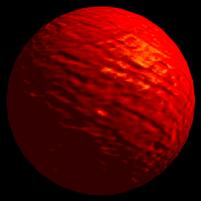Figure 12. Per pixel Phong shading on spheres. Clockwise from upper left, uniform normal and exponent maps, uniform normal map and faceted exponent map, noisy normal and exponent maps with uniform orange specular material, and noisy normal and exponent maps with uniform white specular material.

#### Game Developer Job Board

Browse open positions across the game industry or recruit new talent for your studio